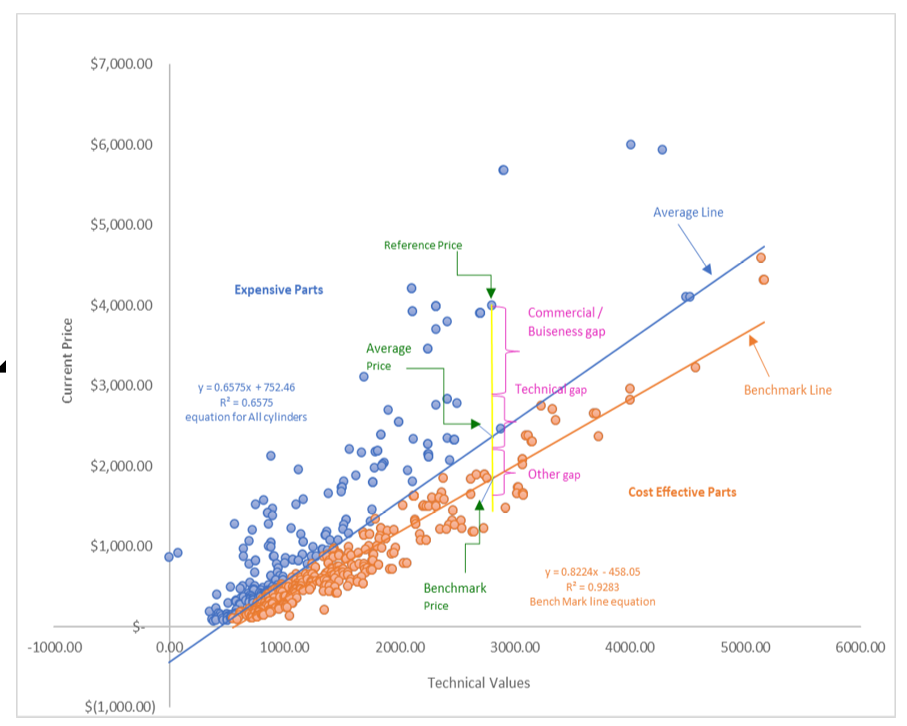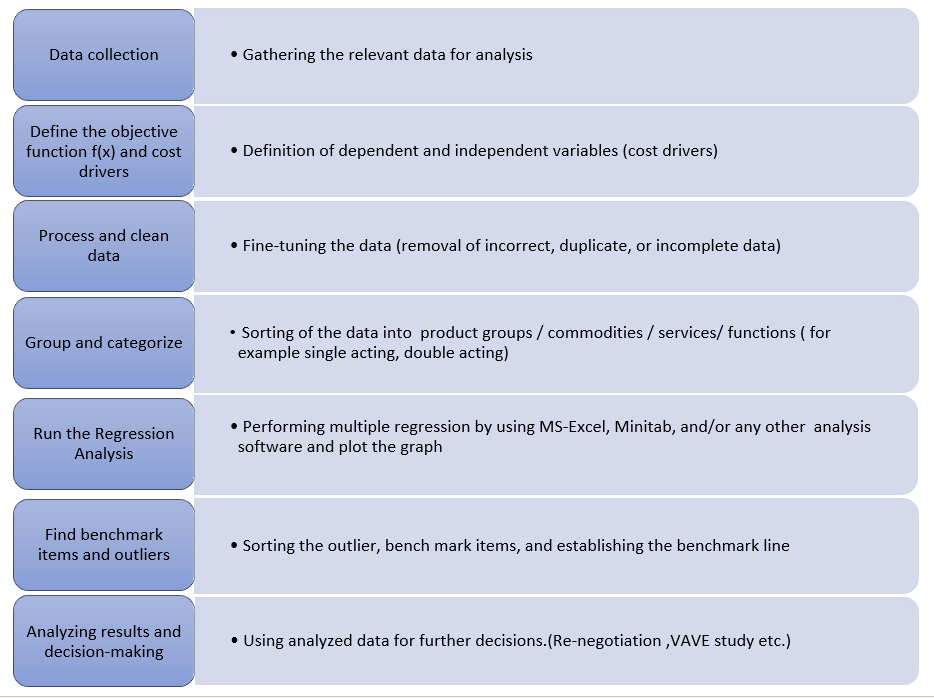Improved pricing decisions by using Regression Analysis] | HCLTech
View Tags

## Improved Pricing decisions by Using Regression Analysis January 07, 2022

For costing engineers, significant work is involved in finding a suitable manufacturing process and the appropriate cost associated with the component or system. When opportunities for in-depth cost analysis come about, the need is to look for the most suitable method to perform the analysis in the shortest possible time. Linear Performance Pricing (LPP) analysis, a regression analysis-based technique, is one such method, which is also one of the simplest and most amenable data analysis and predictive price modeling techniques.

The regression analysis technique is one of the most widely used statistical techniques. Applications of such techniques are widespread in almost every industry possible, including healthcare, finance, marketing, and engineering, to name a few. In the current age of globalization, global procurement and supply chain management have become critical operations. They require quick solutions and enhanced decision-making while handling large and complex data.

Regression modelling, the basis of Linear Performance Pricing is quick, economical and easier than constructing and manipulating real systems.

Regression modeling, the basis of Linear Performance Pricing is quick, economical, and easier than constructing and manipulating real systems (with available information). A regression model represents the essential features of an object, system, or problem. The regression models have the important aspects represented in mathematical form using variables, parameters, and functions, for example, f(x). Analyzing and manipulating the model gives insight into how the real system behaves under various conditions.

The regression analysis (Linear Performance Pricing) technique can be applied in situations where a combination of product variants, manufacturing volumes, and price points present themselves, for each of which large data is available. These situations could arise due to various scenarios and factors, such as part characteristics, globally diverse manufacturing units, supply chain management complexities related to multiple vendors, and price points thereof.

Applicability of such analysis is evidenced on major commodities such as cylinders, hoses, fuel tanks, valves, harnesses, cable, tires, plastics, and the like. One of those commodities explained hereunder is the cylinder. Key factors that drive the decision to select the commodity for analysis are the availability of large data related to part characteristics, manufacturing volumes, and of course, cost data.

In the regression analysis applied to this scenario, a mathematical equation between cost-affecting parameters and the price of the parts is established. In the case of cylinders, cylinder bore diameter, rod diameter, weight, stroke length, pressure rating, and annual quantity, are identified as cost-affecting parameters. After establishing the equation, the price trend is analyzed, and the price of the cylinder is predicted with minimal effort.

The following figure depicts the regression analysis that is carried out for the cylinder commodity.Figure 1 Regression analysis that is carried out for the cylinder commodity.

Process for regression analysis for cylinder commodityTable 1 Process for regression analysis for cylinder commodity

As a result of regression analysis, potential multi-million dollar cost-saving opportunities could be proposed for manufacturing facilities across geographic regions. In this process, a substantially high percentage (up to 25%) of the total number of parts could be outliers (indicating out-of-range or expensive parts) in hydraulic cylinders commodity alone. Hence, only these outlier parts are focused upon separately and detailed should-cost modeling is performed on them. Then these models could be used to negotiate with the supplier to realize savings.

The LPP analysis could also be carried out on other commodities with similar outcomes of price trending for in-range parts and should be used for cost modeling with supplier negotiations for the outlier parts. In effect, considerable savings can be realized with minimal effort.

While doing LPP analysis, a few general assumptions are followed as mentioned below:

• Only a linear relationship between price and cost drivers is considered. Any non-linear relationship is ignored. This is most easily seen in scatter plots shown in the figure above.

• Exceptional outliers due to one-time situations are removed from the data. If not, they may cause an inconsistent variance, abnormality, or other problems with the regression model.

Apart from the above detailed potential cost savings, other possible advantages of regression analysis are listed below:

• Ability to evaluate possible solutions in a quick, easy, and inexpensive way without constructing complex models and experimenting with them.
• Facilitates ‘what if’ analysis. Mathematical models make it relatively easier

to find the optimal solution for a specific model and scenario.

• Helps with prediction and forecasting of the product’s price
• Helps to synthesize the large data with accuracy. It also helps to streamline data analysis and rectify the data.
• A collaborative tool for focused supply cost reduction. Can be applied for supplier analysis and benchmarking.
• Helps to find target parts/outlier parts, which can further be taken up for should costing or value analysis.
• Development of analytical insight for part price assessment and regional price variance.

Possible disadvantages of regression analysis are highlighted below:

• If an important cost driver is not considered/missed out during the LPP analysis, then complete analysis may become incorrect. Hence it is important to find the proper cost driver/parameter.
• Output results depend upon the quality of input data and a large set of data points

Conclusion

The regression model is based on a strong theoretical foundation that demonstrates how technical data and price are related. Based on the experience with regression analysis, historical data of the product and its prices can be effectively utilized for a prediction of the price. It is also useful in supplier validation, negotiation, and target pricing.

References

www.NCSS.com

https://www.thoughtco.com

http://www.uky.edu